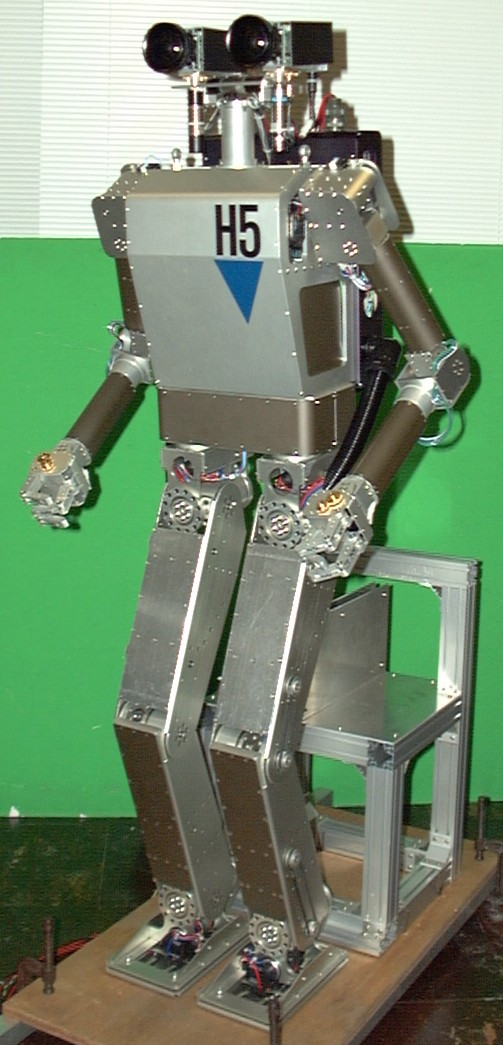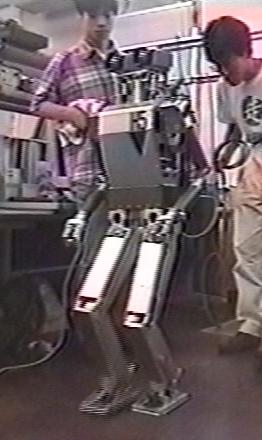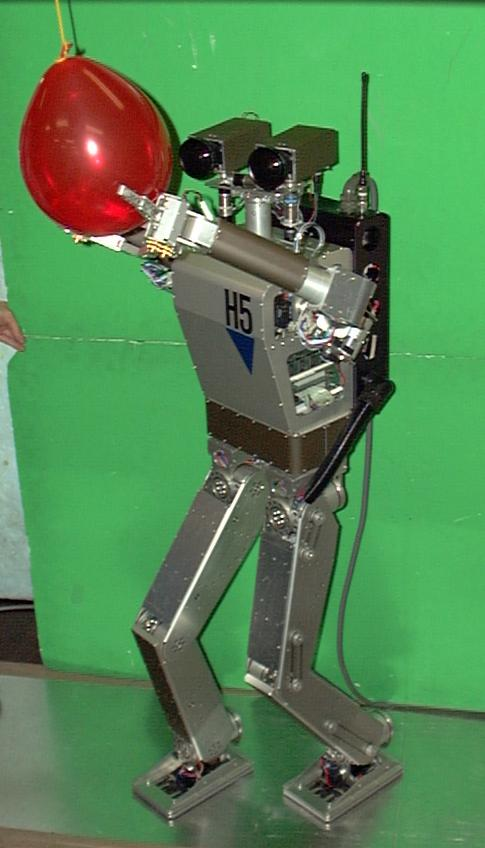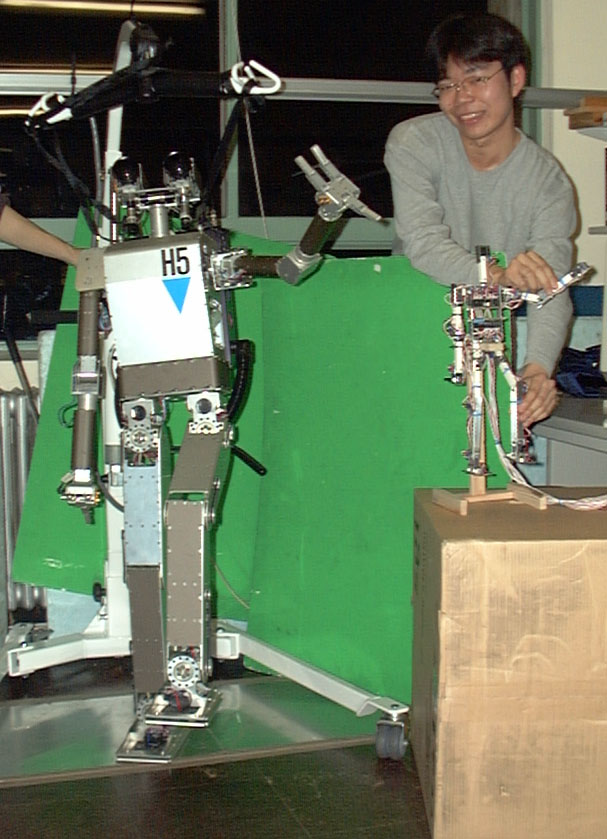# \$B!V#H#5!W%W%m%8%'%/%H(B### \$B35MW(B

\$B!!K\%W%m%8%'%/%H\$G\$O!"?M4V7?%m%\%C%H\$K\$*\$1\$kF0NO3X0BDj\$r9MN8\$7\$?(B \$BF0:n\$N@8@.\$r \$B!!\$3\$l\$^\$G\$K!"4pK\Jb9T!";k3P\$rMQ\$\$\$?5SOS6(D4\$K\$h\$kBP>]J*\$X\$N%"%W%m!<%A!"(B \$B<+M3EYG[CV\$,Aj;w\$J?M7A7?F~NO%G%P%\$%9\$d!"%]%R%^%9%;%s%5\$rMQ\$\$\$F!"(B \$B;Q@*\$r65<(\$9\$k\$3\$H\$K\$h\$kF0NO3X0BDj\$JF0:n@8@.\$N8&5f\$r9T\$C\$F\$-\$F\$\$\$k!#(B

### \$B%R%e!<%^%N%\$%I!V#H#5!W(B

\$B!!A49b(B 1270m\$B!"=ENL(B 33kg\$B\$G!"A4(B30\$B\$N@)8f<+M3EY\$r;}\$D!#(B \$B3FIt\$X\$N<+M3EYG[CV\$O!"5SIt:81&3F#6<+M3EY!"OSIt3F#6<+M3EY!"(B \$B%0%j%C%Q3F#1<+M3EY!"F,It#4<+M3EY\$G\$"\$k!#(B
\$B!!J"It\$K;:6HMQ(BPC\$B5Z\$SF~=PNO%\!<%I\$r\$rEk:\\$7!"%b!<%?\$N%=%U%H%&%(%"(B \$B%5!<%\\$r4^\$`%m%\%C%HA4BN\$N%3%s%H%m!<%k\$r9T\$C\$F\$\$\$k!#(B \$BL5@~(BLAN\$B5Z\$S!"1GA|%H%i%s%9%_%C%?\$rEk:\\$7!"30It\$H\$N%1!<%V%k\$O!"EE8;@~(B \$B\$N\$_\$H\$J\$C\$F\$\$\$k!#L5@~(BLAN\$BMxMQ\$K\$h\$j!"30ItC \$B4pK\Jb9T(B \$BJb9T ZMP(Zero Moment Point)\$B\$N0LCV\$rHsE>E]@-\$N0BDj5,HO\$H\$7\$F!"(B \$B@_7WpJs\$r85\$K!"\$=\$NCM\$,%7%_%e%l!<%7%g%s\$G\$N@_DjCM\$H9g\$&\$h\$&\$K!"(B \$BBN44\$N1?F050F;\$K%U%#!<%I%P%C%/\$r2C\$(\$k!#(B

### \$B;k3P\$rMQ\$\$\$?5SOS6(D4\$K\$h\$kBP>]J*\$X\$N%"%W%m!<%A(B

\$B\$^\$:!"\$\$\$/\$D\$+\$N0BDj\$JBeI=50F;\$N9g@.\$K\$h\$j!"G\$0U\$N0LCV\$XB-\$r(B \$B0lJbF'\$_=P\$9F0NO3XE*0BDj\$J50F;\$r@8@.\$9\$k%7%9%F%`\$r9=C[\$7\$?!#(B
\$BF,It\$N#2Bf\$N%+%a%i\$K\$h\$j!"BP>]J*\$rH/8+DI=>\$7!"%9%F%l%*;k\$K\$h\$j!"(B \$BBP>]J*\$N%m%\%C%H\$+\$i\$N0LCV\$rF1Dj\$7!"\$=\$N0LCV\$K1~\$8\$F!"(B \$BJb\$r?J\$a!";j6a5wN%\$K\$FOS\$G\$D\$+\$`\$3\$H\$K\$h\$j!"J*BN\$X\$N%"%W%m!<%A\$r9T\$C\$?!#(B

### \$BF0NO3X0BDjA4?HF0:n@8@.\$N8&5f(B

\$B\$^\$:!";Q@*\$N65<(%7%9%F%`\$H\$7\$F!"<+M3EYG[CV\$,Aj;w\$G!"Bg\$-\$5\$,(B \$B#3J,\$N#1\$N;Q@*F~NO%G%P%\$%95Z\$S!"%]%R%^%9%;%s%5\$rMQ\$\$\$?;Q@*(B \$BF~NO%7%9%F%`\$N3+H/\$r9T\$C\$?!#F~NO\$5\$l\$?;Q@*Ns\$KBP\$7!"F0NO3XE*(B \$B0BDj\$KJQ7A\$7!"B?MM\$JF0:n\$r \$B \$B!V#H#5!W%W%m%8%'%/%H\$G\$NCN8+\$r85\$K\$7\$F### \$BH/I=O@J8(B

``` \$BD9:e7{0lO:(B, \$B6aLn(B \$BFX(B, \$B@>OF8w0l(B, \$BKL@n(B \$BCN?-(B, \$B?y86CNF;(B, \$B0pMU2m9,(B, \$B0f>eGn0t(B
\$B%@%\$%J%_%C%/%R%e!<%^%N%\$%I(BH5\$B\$N3+H/(B
\$BBh(B16\$B2sF|K\%m%\%C%H3X2q3X=Q9V1i2qM=9F=8(B
pp.837-838, 1998.

 \$B@>OF(B \$B8w0l(B, \$BD9:e(B \$B7{0lO:(B, \$B0pMU(B \$B2m9,(B, \$B0f>e(B \$BGn0t(B
\$B%R%e!<%^%N%\$%I(BH5\$B\$K\$*\$1\$k%@%\$%J%_%C%/F0:n9=C[\$N\$?\$a\$NF0NO3X(B
\$B%7%_%e%l!<%7%g%s4D6-(B,
\$BBh(B16\$B2sF|K\%m%\%C%H3X2q3X=Q9V1i2qM=9F=8(B
pp. 839-840, 1998.

 Ken'ichirou Nagasaka, Masayuki Inaba, Hirochika Inoue,
Walking Pattern Generation for a Humanoid Robot Based on
Proc. of 1999 IEEE Int. Conf. on Systems, Man, and Cybernetics
pp.VI-908 - VI-913, 1999.

 Koichi Nishiwaki, Ken'ichirou Nagasaka, Masayuki Inaba, Hirochika Inoue,
Generation of Reactive Stepping Motion for a Humanoid
by Dynamically Stable Mixture of Pre-designed Motions,
Proc. of 1999 IEEE Int. Conf. on Systems, Man, and Cybernetics
pp.VI-902 - VI-907, 1999.

 \$BD9:e(B \$B7{0lO:(B, \$B0pMU(B \$B2m9,(B, \$B0f>e(B \$BGn0t(B
\$B:GE,8{G[K!\$rMQ\$\$\$??M4V7?%m%\%C%H\$NF0Jb9T%Q%?!<%s@8@.(B,
\$B%m%\%F%#%/%9!&%a%+%H%m%K%/%99V1i2q(B(ROBOMEC '99),
2P1-78-110, 1999.

 \$B@>OF(B \$B8w0l(B, \$BKL@n(B \$BCN?-(B, \$BD9:e(B \$B7{0lO:(B, \$B?y86(B \$BCNF;(B, \$B0pMU(B \$B2m9,(B, \$B0f>e(B \$BGn0t(B,
\$B%@%\$%J%_%C%/%R%e!<%^%N%\$%I!V#H#5!W\$K\$*\$1\$k;k3PO"F07?F'\$_=P\$7F0:n\$Ne(B \$BGn0t(B,
\$BBN440LCV%3%s%W%i%\$%"%s%9@)8f\$rMQ\$\$\$??M4V7?%m%\%C%H\$NJb9T0BDj2=(B,
\$BBh(B17\$B2sF|K\%m%\%C%H3X2q3X=Q9V1i2qM=9F=8(B,
pp.1193-1194, 1999.

 \$BD9:e(B \$B7{0lO:(B, \$B0pMU(B \$B2m9,(B, \$B0f>e(B \$BGn0t(B,
\$BF0NO3XF0:nJQ49%U%#%k%?\$rMQ\$\$\$??M4V7?%m%\%C%H\$NA4?H9TF0@_7W(B,
\$BBh(B17\$B2sF|K\%m%\%C%H3X2q3X=Q9V1i2qM=9F=8(B,
pp.1207-1208, 1999.

 \$BKL@n(B \$BCN?-(B, \$BD9:e(B \$B7{0lO:(B, \$B@>OF(B \$B8w0l(B, \$B0pMU(B \$B2m9,(B, \$B0f>e(B \$BGn0t(B,
\$B0dEAE*%"%k%4%j%:%`\$rMQ\$\$\$??M4V7?%m%\%C%H\$N5SOS6(D4F0E*5/N)F0:n\$N@8@.(B,
\$BBh(B17\$B2sF|K\%m%\%C%H3X2q3X=Q9V1i2qM=9F=8(B,
pp.1191-1192, 1999.

 \$B?y86(B \$BCNF;(B, \$BD9:e(B \$B7{0lO:(B, \$B0pMU(B \$B2m9,(B, \$B0f>e(B \$BGn0t(B,
\$B?M4V7?%m%\%C%H\$N4X@a%H%k%/:G>.2=\$K4p\$E\$/L\I8#Z#M#P50F;\$N@8@.(B,
\$BBh(B17\$B2sF|K\%m%\%C%H3X2q3X=Q9V1i2qM=9F=8(B
pp.1189-1190, 1999.
```